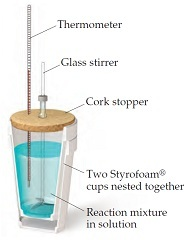# Problem: When a 4.25-g sample of solid ammonium nitrate dissolves in 60.0 g of water in a coffee-cup calorimeter, the temperature drops from 22.0 oC to 16.9 oC.You may want to reference (Pages 179 - 183) Section 5.5 while completing this problem.Calculate ΔH (in kJ/mol NH4NO3) for the solution process:NH4NO3(s) → NH4+(aq) + NO3-(aq)Assume that the specific heat of the solution is the same as that of pure water.Is this process endothermic or exothermic?

###### FREE Expert Solution

We are asked to calculate ΔH (in kJ/mol NH4NO3) for the solution process:
NH4NO3(s) → NH4+(aq) + NO3-(aq)

Calculate heat released by water.

q = -1280.3 J

100% (296 ratings)###### Problem Details

When a 4.25-g sample of solid ammonium nitrate dissolves in 60.0 g of water in a coffee-cup calorimeter, the temperature drops from 22.0 oC to 16.9 oC.You may want to reference (Pages 179 - 183) Section 5.5 while completing this problem.

Calculate ΔH (in kJ/mol NH4NO3) for the solution process:
NH4NO3(s) → NH4+(aq) + NO3-(aq)

Assume that the specific heat of the solution is the same as that of pure water.

Is this process endothermic or exothermic?